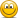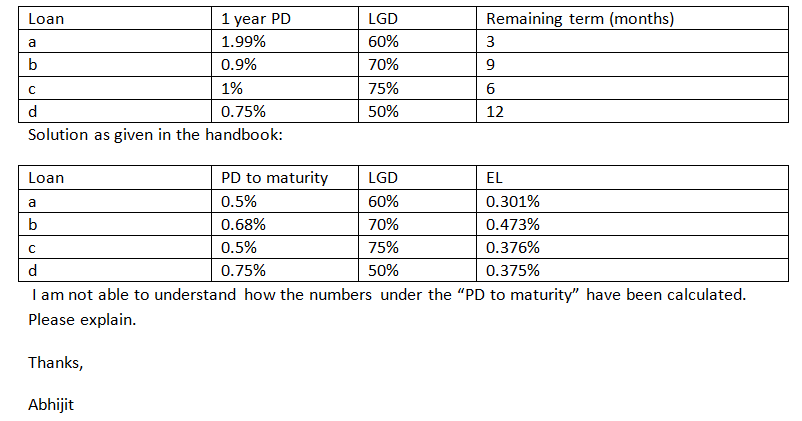probability of default annualized vs no of days for loan

riskybiz

New Member
hi guys, i am struggling with this. say i am given an annual probabilty of default for a company going insolvent as 0.02. so 2%.

say this client then takes out a 100k , 150 day loan on jan 1st 2018, what is predicted default amount?

am i right in thinking you can model this as bernoulli ? i am not sure how to calcualte this. do i need to find default rate per month first using p_annual = p_month^12 ?? i could rearrange this to find p_month then do (100/days in month)*p_month

Matthew Graves

Active Member
Subscriber
Maybe take a hazard rate type approach? A little bit rusty on this so may be wrong! I'm sure someone will correct meIf probability of default in a year is 2% then probability of survival must be 98%. Use this to obtain the hazard rate/default intensity:

$$P(S)=e^{-Ht}$$
$$\therefore H=-\frac{ln(P(S))}{t}$$

Using t=365 and P(S)=0.98 gives H=0.0000553.
Now, using the first formula obtain the probability of surviving until T=150:

$$P(S_{150})=e^{-0.0000553*150}=0.992$$

Which gives probability of defaulting between t=0 and t=150 as 1-0.992=0.83%

We're obviously making the assumption here that the hazard rate is constant which is not usually the case.

riskybiz

New Member
many thanks in what situations is using the hazard appraoch suitable?

Matthew Graves

Active Member
Subscriber
Typically used for pricing credit sensitive instruments such as corporate bonds, CDS etc. Usually you would derive a hazard rate curve from observable market data like bond quotes and use it in conjunction with other rates curves to price the instrument or determine sensitivities.

riskybiz

New Member
thanks... another approach would be to do loan amount * (loan duration/365) * probabilty of default in 1 year . e.g. 100k*(180/365)*0.01, but does this make sense? because then we are assuming a linear relationship on how probabilility changes over year. so e.g. we are saying that half way through year the probability is 0.005.. ?

David Harper CFA FRM

David Harper CFA FRM
Staff member
Subscriber
Hi @riskybiz You can treat the default pattern as monthly which is along the lines of how mortgages/MBS are treated via conditional prepayment rate (CPR) and single month mortality (SMM). CPR is the default rate per annum such that that annualized survival = (1-CRP) = (1-SMM)^12 and therefore the SMM = 1 - (1-CPR)^(1/12). Here is an actual FRM question https://forum.bionicturtle.com/threads/lowest-credit-risk.10473/ i.e.,
Hi David,
This is a problem from FRM handbook page 594:
Which of the following loans has the lowest credit risk?... so under a monthly frequency, if the annualized PD is 2.0%, then the 5-month cumulative PD = 1 - (1 - 0.020)^(5/12) = 0.8382%. There's no reason you can't do this daily with 150-day cumulative PD = 1 - (1 - 0.020)^(150/360) = 0.8340%.

Where k = periods per year (eg., k = 12 if monthly), the general form is Δt-period cumulative PD = 1 - (1 - PD_annualized)^(Δt/k). This is the same thing @Matthew Graves is showing you but in discrete time so it's a little clunkier than his. I hope that's helpful,

Last edited:

riskybiz

New Member
thank you for this. but why would it make sense to use this method to my problem since when they take a loan out they immediately use the money. i wonder if this affects the calcalation in anyway. since if they use loan immediately surely the probability of default is higher in short term rather than as days go by. also by using the method above are we not then assuming a liner relationship?

Last edited:

David Harper CFA FRM

David Harper CFA FRM
Staff member
Subscriber
Hi @riskybiz Yes, my assumption (like @Matthew Graves 's) is "linear" or like him, I'd probably prefer to say that it's a constant assumption. It doesn't need to be that way, but for myself, if we are talking about "scaling down" from an annual PD to a monthly (or 150 out of 250 days), lacking data, it seems like a fine assumption; e.g., I'm not sure why default is more likely in first month than the third or fifth month (again, lacking data ...). In regard to using the loan immediately, the default probability (PD) typically does assume there is an exposure: it assumes the principal has been lended.

We use expected loss (EL) = exposure at default (EAD) × default probability (PD or expected default frequency, EDF) × loss given default (LGD); i.e., EL = EAD × PD × LGD.

The EAD is definitely a parameter than can be adjusted; but if $150K is lent, with principal at risk, I think EAD = 150K is a basic assumption. On the other hand, if a bank has a line of credit or Commitment to lend$150K (the client has not drawn down) then the typical approach is that EAD = OS + (COM - OS)*UGD. For example, bank has a commitment (COM) of $150K but client has only drawn (OS =)$50K such that maybe EAD = 50 + (150 - 50)*65% = \$115; in this example, the 50 is fully exposed but only 65% of the "unused commitment" of 100 is counted toward exposure (UGD = usage given default which treats the unused commitment as a contingent claim/credit option). It's just to show that we can adjust the EAD. Thanks,

Last edited: